# viscosity

(redirected from Viscousity)
Also found in: Dictionary, Thesaurus, Medical.
Related to Viscousity: Viscosity index

## viscosity,

resistance of a fluid to flow. This resistance acts against the motion of any solid object through the fluid and also against motion of the fluid itself past stationary obstacles. Viscosity also acts internally on the fluid between slower and faster moving adjacent layers.

All fluids, i.e., all liquids and gases, exhibit viscosity to some degree. Viscosity may be thought of as fluid frictionfriction,
resistance offered to the movement of one body past another body with which it is in contact. In certain situations friction is desired. Without friction the wheels of a locomotive could not "grip" the rails nor could power be transmitted by belts.
..... Click the link for more information.
, just as the friction between two solids resists the motion of one over the other but also makes possible the acceleration of one relative to the other (e.g., the friction between the wheels of an automobile and a highway), so viscosity resists the motion of a solid through a fluid but also makes it possible for a propeller or other device to accelerate the solid through the fluid.

### The Velocity Gradient

When a fluid is moving through a pipe or a solid object is moving through a fluid, the layer of fluid in contact with the sides of the pipe or the surface of the object tends to be in the same state of motion as the object with which it is in contact; that is, the layer of fluid along the side of the pipe is at rest, while that in contact with the moving object is carried along at the same velocity as the object. If the difference in velocity between the fluid at the sides of the pipe and that at the center, or between the moving object and the fluid through which it is moving, is not too great, then the fluid flows in continuous, smooth layers; that is, the flow is laminar.

The difference in velocity between adjacent layers of the fluid is known as a velocity gradient and is given by v/x, where v is the velocity difference and x is the distance between the layers. To keep one layer of fluid moving at a greater velocity than the adjacent layer, a force F is necessary, resulting in a shearing stress F/A, where A is the area of the surface in contact with the layer being moved.

### The Coefficient of Viscosity

The ratio of the shearing stress to the velocity gradient is a measure of the viscosity of the fluid and is called the coefficient of viscosity η, or η=Fx/Av. The cgs unit for measuring the coefficient of viscosity is the poise. Experiments have shown that the coefficient of viscosity of liquids decreases with increasing temperature, while the coefficient of viscosity of gases increases with increasing temperature. In liquids an increase in temperature is associated with the weakening of bonds between molecules; since these bonds contribute to viscosity, the coefficient is decreased. On the other hand, intermolecular forces in gases are not as important a factor in viscosity as collisions between the molecules, and an increase in temperature increases the number of collisions, thus increasing the coefficient of viscosity. A striking result of the kinetic theory of gases is that the viscosity of a gas is independent of the density of a gas. Viscosity is the principal factor resisting motion in laminar flow. However, when the velocity has increased to the point at which the flow becomes turbulent, pressure differences resulting from eddy currents rather than viscosity provide the major resistance to motion.

The Columbia Electronic Encyclopedia™ Copyright © 2013, Columbia University Press. Licensed from Columbia University Press. All rights reserved. www.cc.columbia.edu/cu/cup/

## Viscosity

The material property that measures a fluid's resistance to flowing. For example, water flows from a tilted jar more quickly and easily than honey does. Honey is more viscous than water, so although gravity creates nearly the same stresses in honey and water, the more viscous fluid flows more slowly.

The viscosity can be measured where the fluid of interest is sheared between two flat plates which are parallel to one another (see illustration). This is known as planar Couette flow. The shear stress is the ratio of the tangential force F needed to maintain the moving plate at a constant velocity V to the plate area A. The shear flow created between the plates has the velocity profile given by Eq. (1),

(1)where vx is the velocity parallel to the plates at a perpendicular distance y above the stationary plate. The coefficient γ &dot;, called the velocity gradient or shear rate, is given by V/δ, where δ is the distance between the plates. It is expected that the shear stress increases with increasing shear rate but that the ratio of these two quantities depends only on the fluid between the plates. This ratio is used to define the shear viscosity, &eegr;, as in Eq. (2).
(2)The shear viscosity may depend on temperature, pressure, and shear rate.

Isaac Newton is credited with first suggesting a model for the viscous property of fluids in 1687. Newton proposed that the resistance to flow caused by viscosity is proportional to the velocity at which the parts of the fluid are being separated from one another because of the flow. Although Newton's law of viscosity is an empirical idealization, many fluids, such as low-molecular-weight liquids and dilute gases, are well characterized by it over a large range of conditions. However, many other fluids, such as polymer solution and melts, blood, ink, liquid crystals, and colloidal suspensions, are not described well by Newton's law. Such fluids are referred to as non-newtonian.

For planar Couette flow, Newton's law of viscosity is given mathematically by

(3)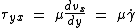Eq. (3), where is the shear stress, and μ, a function of temperature and pressure, is the coefficient of viscosity or simply the viscosity. Therefore, by comparing Eqs. (2) and (3) the shear viscosity is equal to the coefficient of viscosity (that is, η = μ) for a newtonian fluid. Because of this re­lation the shear viscosity is also often referred to as the viscosity. However, it should be clear that the two quantities are not equivalent; μ is a newtonian-model parameter, which varies only with temperature and pressure, while &eegr; is a more general material property which may vary nonlinearly with shear rate. See Fluid flow, Newtonian fluid

Viscosity conversions
Unit poise cp Pa · s m/(ft · s) lbf · s/ft2
1 poise* 1 100 0.1 6.72 × 10-2 2.089 ×10-3
1 centipoise 0.01 1 0.001 6.72 × 10-4 2.089 × 10-5
1 pascal-second 10 1000 1 0.672 2.089 × 10-2
1 lbm/(ft · s) 14.88 1488 1.488 1 3.108 × 10-2
1 lbf · s/ft2 478.8 4.788 × 104 47.88 32.17 1
*1 poise = 1 dyne · s/cm2 = 1 g/(cm · s). 1 Pa · s = 1 kg/(m · s).

From Eqs. (2) and (3), the units of viscosity are given by force per area per inverse time. If in planar Couette flow, for example, 1 dyne of tangential force is applied for every 1 cm2 area of plate to create a velocity gradient of 1 s-1, then the fluid between the plates has a viscosity of 1 poise (=1 dyne · s/cm2). Several viscosity units are in common use (see table). Comparison of the viscosities of different fluids demonstrates some general trends. For example, the viscosity of gases is generally much less than that of liquids. Whereas gases tend to become more viscous as temperature is increased, the opposite is true of liquids. Other data also show that increasing pressure tends to increase the viscosity of dense gases, but pressure has only a small effect on the viscosity of dilute gases and liquids.

Whereas dilute gas molecules interact primarily in pairs as they collide, molecules in the liquid phase are in continuous interaction with many neighboring molecules. The concepts of velocity and mean free path have little meaning for liquids. It is clear, however, that increasing temperature increases the mobility of molecules, thus allowing neighboring molecules to more easily overcome energy barriers and slip past one another. Such arguments lead to an exponential relation for the dependence of viscosity on temperature.

Many non-newtonian fluids not only exhibit a viscosity which depends on shear rate (pseudoplastic or dilantant) but also exhibit elastic properties. These viscoelastic fluids require a large number of strain-rate-dependent material properties in addition to the shear viscosity to characterize them. The situation can become more complex when the material properties are time dependent (thixotropic or rheopectic). Fluids that are nonhomogeneous or nonisotropic require even more sophisticated analysis. The field of rheology attempts to deal with these complexities. See Rheology

McGraw-Hill Concise Encyclopedia of Physics. © 2002 by The McGraw-Hill Companies, Inc.
The following article is from The Great Soviet Encyclopedia (1979). It might be outdated or ideologically biased.

## Viscosity

(internal friction), the property of fluids (liquids and gases) of resisting the displacement of one part of the substance relative to another. The viscosity of solids has a number of specific features and is usually considered separately.

The basic law of viscous flow was established by I. Newton in 1687: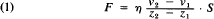where F is the tangential force, which causes the displacement of the layers of a liquid or gas relative to each other; S is the area of the layer along which the displacement occurs; and (v2-v1)/Z2-Z1) is the gradient of the flow velocity (the rate of change from layer to layer)—in other words, the dis-placement velocity (see Figure 1). The proportionality constant η is called the dynamic-viscosity coefficient, or simply the viscosity. It characterizes quantitatively the resistance of the liquid or gas to displacement of its layers. The reciprocal of viscosity, Φ = 1/η, is called the fluidity.Figure 1. Diagram of uniform displacement (viscous flow) of a layer of liquid enclosed between two solid plates of area S. The lower plate (A) is immovable, and the upper plate (B) moves with constant velocity v0 under the influence of a tangential force F; v(z) is the dependence of the velocity of the layer on its distance z from the immovable plate: Δx0 is the value of the initial displacement of the liquid.

According to formula (1), the viscosity is numerically equal to the tangential force—Ps = F/S (per unit area)—required to maintain a velocity difference equal to 1 between two parallel layers of a liquid or gas whose distance from each other is equal to 1. From this definition it follows that the unit of viscosity has the dimensions newton · sec/m2 in the International System of Units; in the centimeter-gram-second system it has the dimensions gram/(cnvsec) (poise), where 1 poise = 0.1 N-sec/m2. The so-called kinematic viscosity, v = η/P where p is the density of the liquid or gas, is often considered along with the dynamic viscosity η. The units of kinematic viscosity are m2/sec and cm2/sec (stokes), respectively. Viscometers are used to determine the viscosity of gases and liquids.

Under conditions of steady-state laminar flow at constant temperature, the viscosity of gases and normal liquids (so-called Newtonian liquids) is a constant that does not depend on the velocity gradient. The viscosity values of some liquids and gases are given in Table 1.

Table 1. Viscosity values of some fluids (liquids and gases)
η at 20° C
(10-3 N·sec/m2, or centipoises)
Hydrogen ...............0.0088
Nitrogen ...............0.0175
Oxygen ...............0.0202
Water ...............1.002
Ethyl alcohol ...............1.200
Mercury ...............1.554
Glycerin ...............~1,500

The viscosity of molten metals is of the same order as ordinary liquids (see Figure 2). Liquid helium has peculiar viscous properties. At 2.19° K and normal pressure it passes into a superfluid state, in which its viscosity is zero.

Viscosity is an important physicochemical property of matter. The viscosity value must be considered in pumping liquids and gases through tubes such as petroleum and gas pipelines. The viscosity of molten slag is critical in the blast-furnace and open-hearth processes. The viscosity of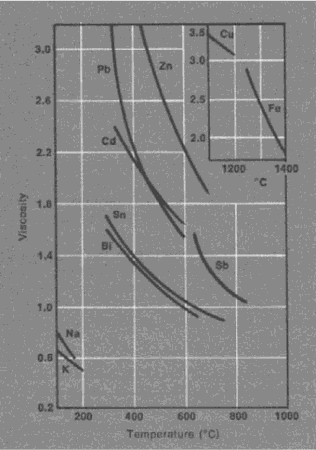Figure 2. Viscosity of some molten metals (in centipoises)

molten glass determines its method of production. Viscosity is often a criterion of readiness or quality of products or intermediate products, since it is closely associated with the structure of a substance and reflects the physicochemical changes in the material that occur during technological processes. The viscosity of oils is of great importance in calculating the lubrication of machines and mechanisms.

Kinetic molecular theory explains viscosity by the motion and interaction of molecules. In gases the distance between molecules is much greater than the radius of action of molecular forces; therefore, the viscosity of gases is chiefly determined by molecular motion. A constant exchange of molecules occurs between layers of a gas that are moving relative to one another; this exchange is due to their continuous chaotic (thermal) motion. The transition of molecules from one layer to the next layer, which is moving at a different velocity, leads to the transfer of a certain amount of motion from one layer to the other. As a result, slow layers speed up and faster layers slow down. The action of an external force F, which balances viscous drag and which maintains steady-state flow, is completely converted to heat.

The viscosity of a gas does not depend on its density (pressure), since when the gas is compressed the total number of molecules that pass from layer to layer increases, but each molecule penetrates less deeply into the neighboring layer and transmits less motion (Maxwell’s law). The viscosity of ideal gases is given in terms of kinetic molecular theory by the relation

(2) η=1/3mnūλ

where m is the mass of the molecule, n is the number of molecules per unit volume, ū is the average molecular velocity, and λ is the free path length of a molecule between collisions with other molecules. Since ū increases with an in-crease in temperature T (λ also increases somewhat), the viscosity of a gas increases upon heating, proportional to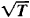The concept of viscosity becomes meaningless for very rarefied gases.

In liquids, where the distance between molecules is much smaller than in gases, viscosity is primarily due to inter-molecular interaction, which limits molecular mobility. In a liquid the molecule can penetrate into a neighboring layer only upon the formation in the layer of a cavity that is large enough to hold the molecule. The so-called activation energy of viscous flow is expended in forming a cavity (“loosening” the liquid). The activation energy decreases with an increase in temperature and a reduction in pressure. This is one of the reasons for the sharp decrease of the viscosity of liquids with an increase in temperature (see Figure 3) and its increase at high pressures. An increase in pressure to several thousand atmospheres increases η by tens and hundreds of times.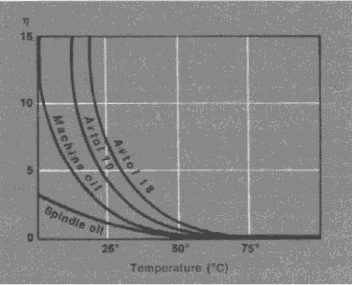Figure 3. Change with temperature of the viscosity of some lubricating oils (η is given in poises)

Because of the inadequate development of the theory of the liquid state, a strict theory of the viscosity of liquids has not yet been created. A number of empirical and semiempirical viscosity formulas, which adequately reflect the dependence of viscosity of some classes of liquids and solutions on temperature, pressure, and chemical composition, are widely used in practice.

The viscosity of liquids depends on the chemical structure of the liquid’s molecules. In series of similar chemical compounds—for example, saturated hydrocarbons, alcohols, and organic acids—viscosity varies regularly: it increases with an increase in molecular weight. The high viscosity of lubricating oils is explained by the presence of cycles in their molecules. Two liquids of different viscosity that do not react with each other when mixed will have the average of their viscosity values in a mixture. If a chemical compound is formed upon mixing, the viscosity of the mixture may be dozens of times greater than the viscosities of the original liquids. The use of viscosity measurements as a method of physicochemical analysis is based on this.

The emergence in liquids (disperse systems or solutions of polymers) of spatial structures formed by the linking of particles or macromolecules causes a sharp increase in viscosity. In the flow of a “structurized” liquid, the action of an external force is consumed not only in surmounting the true (Newtonian) viscosity but also in breaking down the structure.

For normal viscous liquids there is a direct proportionality between the pressure p and the amount of liquid Q passing through a capillary per unit time (Poiseuille’s law). The flow of structurized liquids is not governed by this law; for such liquids, the Q-p curves are convex toward the pressure axis (see Figure 4), which is explained by the variability of η. Abnormal viscosity, which is characteristic for structurized liquid systems, is typical for vital biological mediums—for example, cytoplasm and blood.

M. P. VOLAROVICH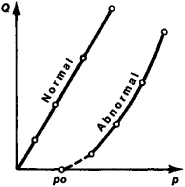Figure 4. Dependence on pressure p of the amount of liquid Q that passes through a capillary in 1 sec for normal (Newtonian) and abnormal (non-Newtonian) liquids

Viscosity of biological mediums. The viscosity of biological mediums is determined in most cases by structural viscosity. The viscosity of the liquid contents of the cell—cytoplasm— is associated with the structure of the biopolymers and sub-cellular formations that make up the cell, which causes deviations (thixotropy) of the viscous flow from the Newtonian law for normal liquids. The methods of measuring the viscosity of biological mediums include observation of the displacement velocity of granules upon centrifugation or of iron filings in a magnetic field, or measurement of the average displacement of Brownian particles. The absolute viscosity of cytoplasm varies from 2 to 50 centipoises (1 centipoise = 10-3 N·sec/m2); it varies in different parts of the cell and at different periods of the cellular cycle. When the temperature is reduced to below 12°-15° C or raised above 40°-50° C, the viscosity of cytoplasm increases. Irradiation at first reduces the viscosity and then, with in-creased dosage, increases it.

The viscosity of amniotic fluid, lymph, and blood plasma is described with sufficient accuracy by the Newtonian law of viscous flow; it is investigated in capillary or cylindrical viscometers. Blood is a non-Newtonian liquid, since it contains structurized components—proteins and blood cells; its viscosity in humans is normally 4-5 centipoises, and in pathological conditions it varies from 1.7 to 22.9 centipoises, which is reflected in the erythrocyte sedimentation reaction.

N. N. FIRSOV

### REFERENCES

Hatschek, E. Viazkost’ zhidkostei, 2nd ed. Moscow-Leningrad, 1935. (Translated from English.)
Trudy soveshchaniia po viazkosti zhidkostei i kolloidnykh rastvorov, vols. 1-3. Moscow-Leningrad, 1941-45.
Frenkel’, la. I. Kineticheskaia teoriia zhidkostei. Moscow-Leningrad, 1945.
Fuks, G. I. Viazkost’ i plastic hnost’ nefteproduktov. Moscow, 1956.
Golubev, I. F. Viazkost’ gazov i gazovykh smesei. Moscow, 1959.
Spravochnik khimika, 2nd ed., vol. 1. Leningrad-Moscow, 1963.
Rukovodstvo po tsitologii, vols. 1-2. Moscow-Leningrad, 1965-66.
Heilbrunn, L. V. The Viscosity of Protoplasm. Vienna, 1958.
The Great Soviet Encyclopedia, 3rd Edition (1970-1979). © 2010 The Gale Group, Inc. All rights reserved.

## viscosity

[vi′skäs·əd·ē]
(fluid mechanics)
The resistance that a gaseous or liquid system offers to flow when it is subjected to a shear stress. Also known as flow resistance; internal friction.
McGraw-Hill Dictionary of Scientific & Technical Terms, 6E, Copyright © 2003 by The McGraw-Hill Companies, Inc.

## viscosity

The internal frictional resistance exhibited by a fluid in resisting a force which tends to cause the liquid to flow.
McGraw-Hill Dictionary of Architecture and Construction. Copyright © 2003 by McGraw-Hill Companies, Inc.

## viscosity

Physics
a. the extent to which a fluid resists a tendency to flow
b. a measure of this resistance, equal to the tangential stress on a liquid undergoing streamline flow divided by its velocity gradient. It is measured in newton seconds per metre squared.
Collins Discovery Encyclopedia, 1st edition © HarperCollins Publishers 2005
Site: Follow: Share:
Open / Close